• 首字母+其余字符串的全排列，直到其余字符串长度为1返回。 最终利用list(set(lst))对lst去重。 理解简单，书写便捷，缺点是耗时较久。 class Solution: #递归 def permutation(self, s: str): i...

一、题目描述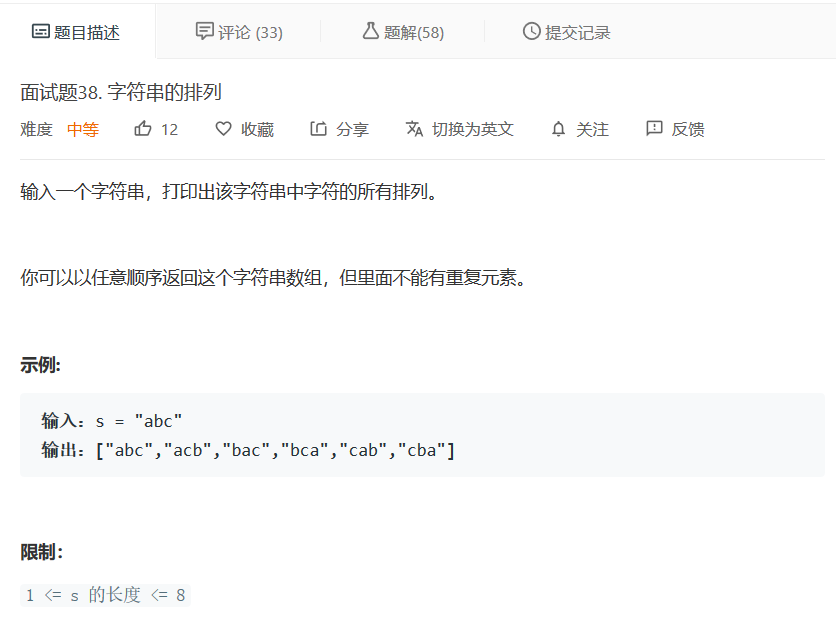二、思路以及代码

递归思路：

分解字符串，将其视为第一个字符和其余字符的组合，利用这个函数反复得到
首字母+其余字符串的全排列，直到其余字符串长度为1返回。
最终利用list(set(lst))对lst去重。
理解简单，书写便捷，缺点是耗时较久。

class Solution:
#递归
def permutation(self, s: str):
if len(s)==1:
return [s]
lst=[]
for index in range(len(s)):
rets=self.permutation(s[:index]+s[index+1:])
for ret in rets:
lst.append(s[index]+ret)
return list(set(lst))

循环思路

参考了“he___jia”的题解，笔者手写代码。
主要思路是：
从一个字符开始，每轮循环增加一个字符，
在之前字符全排列的基础上逐空插入下一个字符，最终得到多一个字符的全排列，循环下去，得到所有字符的全排列。

class Solution:
#循环
def permutation(self, s: str):
rets=set(s)
index,l=1,len(s)
#循环开始
while(index<len(s)):
temp=set()
for ret in rets:
for j in range(len(ret)+1):
string=ret[:j]+s[index]+ret[j:]
index+=1
rets=temp
return list(rets)

三、执行用时比较

笔者手写的递归740ms，循环76ms（原循环题解作者为40ms，膜拜）。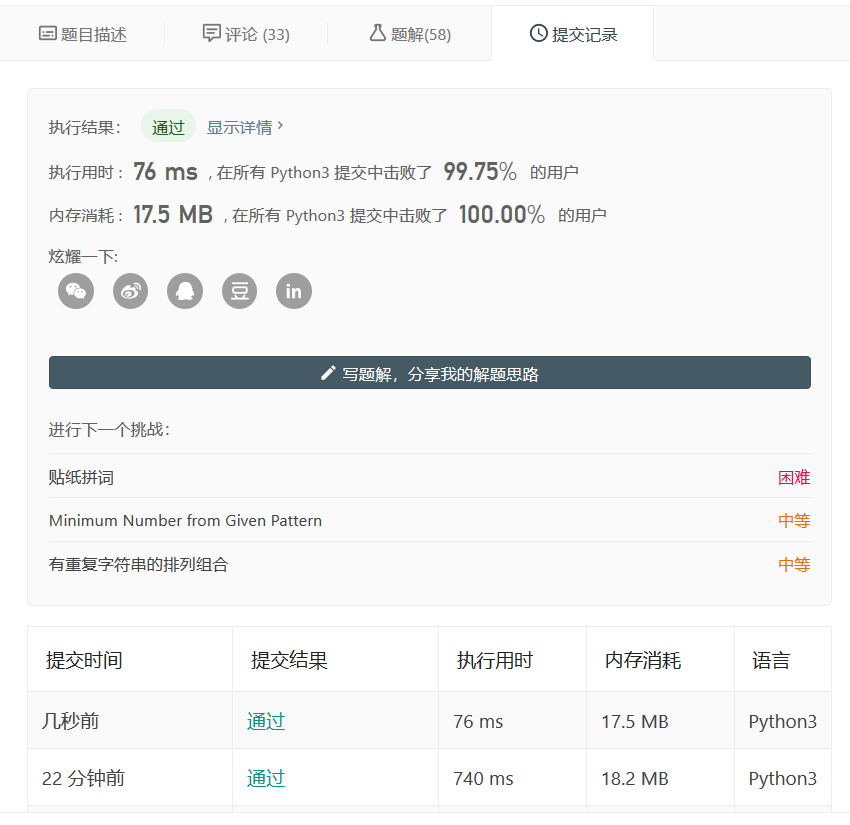展开全文• 567. 字符串排列 给你两个字符串 s1 和 s2 ，写一个函数来判断 s2 是否包含 s1 的排列。 换句话说，s1 的排列之一是 s2 的 子串 。 示例 1： 输入：s1 = "ab" s2 = "eidbaooo" 输出：true 解释：s2 包含 s1 的排列...

567. 字符串的排列

给你两个字符串 s1 和 s2 ，写一个函数来判断 s2 是否包含 s1 的排列。

换句话说，s1 的排列之一是 s2 的 子串 。

示例 1：

输入：s1 = "ab" s2 = "eidbaooo"
输出：true
解释：s2 包含 s1 的排列之一 ("ba").

示例 2：

输入：s1= "ab" s2 = "eidboaoo"
输出：false

提示：

• 1 <= s1.length, s2.length <= 104
• s1 和 s2 仅包含小写字母

来源：力扣（LeetCode）
链接：https://leetcode-cn.com/problems/permutation-in-string。

• 先将s1字符串中每个字符出现的次数存下来，再每次在s2中遍历相同长度的字符串，比较每个字符出现的次数。
class Solution {
public:
bool checkInclusion(string s1, string s2) {
std::vector<int> ch1_counts(26);
for (auto &ch : s1) {
ch1_counts[ch - 'a']++;
}
int length = s1.size();
if (length > s2.size()) {
return false;
}
std::vector<int> ch2_counts(26);
int left = 0;
int right = 0;
for (right = 0; right < length; ++right) {
ch2_counts[s2[right] - 'a']++;
}
if (ch1_counts == ch2_counts) {
return true;
}
for (right; right < s2.size(); ++right) {
ch2_counts[s2[left++] - 'a']--;
ch2_counts[s2[right] - 'a']++;
if (ch1_counts == ch2_counts) {
return true;
}
}
return false;
}
};

• 优化, 可将ch2_counts省略掉：每次都只有一个字符的变化，没必要将两个完整的数组进行比较。s1可以使用–，s2使用++，sum == 0则表示找到字符串；每一个字符变化的时候，只需要统计这一个字符变化时sum的值变换情况即可。
展开全文c++ 滑动窗口 字符串
• 567. 字符串排列 s1字符串26小写字母统计 s2连续查找抵消s1统计 某个字符ch1[k] < 0时按照s2从left开始回复ch1[k] s2连续包含,匹配个数为s1长度i - left + 1 == n，返回true class Solution { public ...

567. 字符串的排列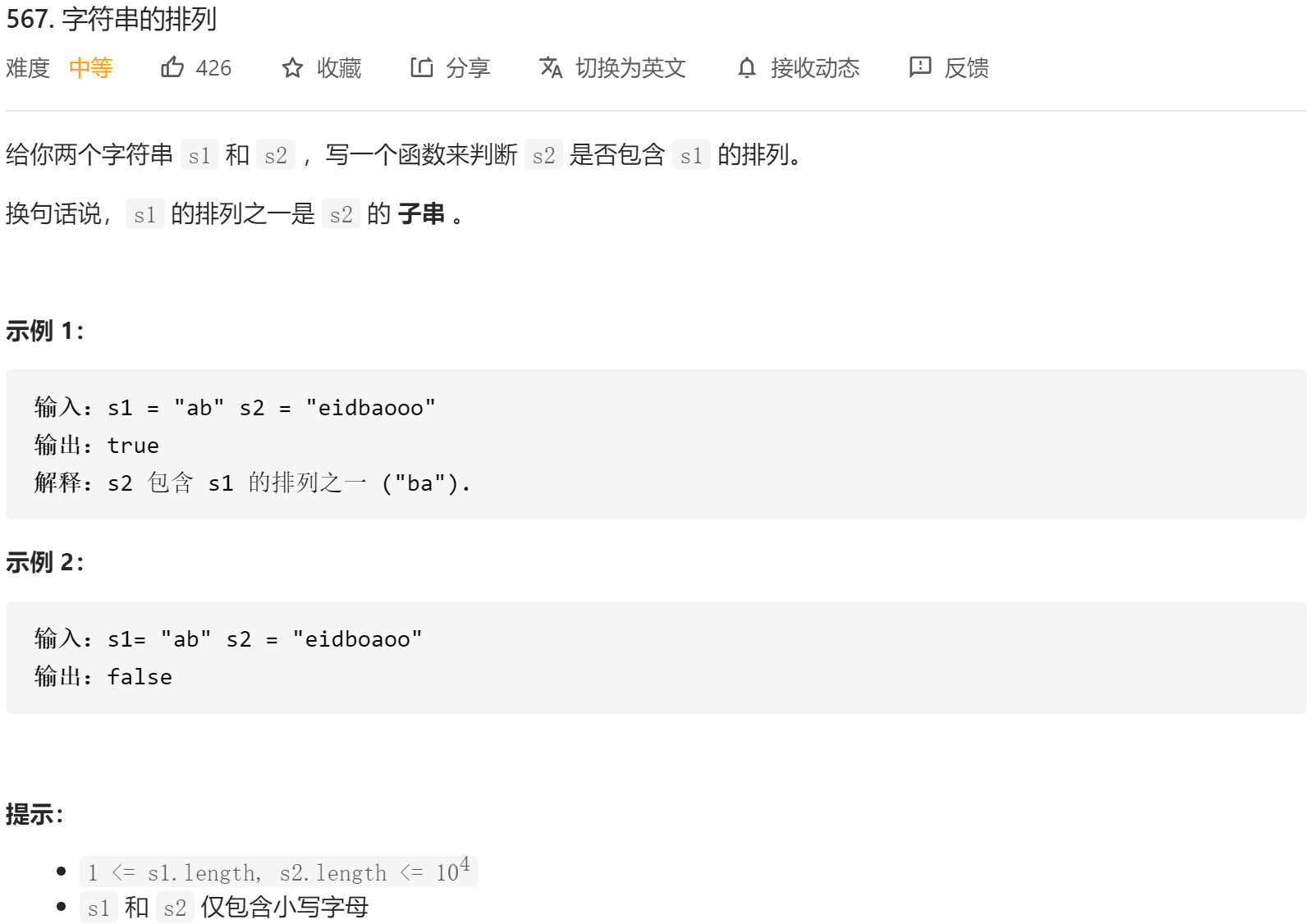• s1字符串26小写字母统计
• s2连续查找抵消s1统计
• 某个字符ch1[k] < 0时按照s2left开始回复ch1[k]
• s2连续包含,匹配个数为s1长度i - left + 1 == n，返回true
class Solution {
public boolean checkInclusion(String s1, String s2) {
int n = s1.length();
int m = s2.length();
if (n > m) {
return false;
}

int[] ch1 = new int;

for (int i = 0; i < n; i++) {
int k = s1.charAt(i) - 'a';
++ch1[k];
}

int left = 0;
for (int i = 0; i < m; i++) {
int k = s2.charAt(i) - 'a';
--ch1[k];
while (ch1[k] < 0) {
++ch1[s2.charAt(left) - 'a'];
++left;
}
if (i - left + 1 == n) {
return true;
}
}

return false;
}
}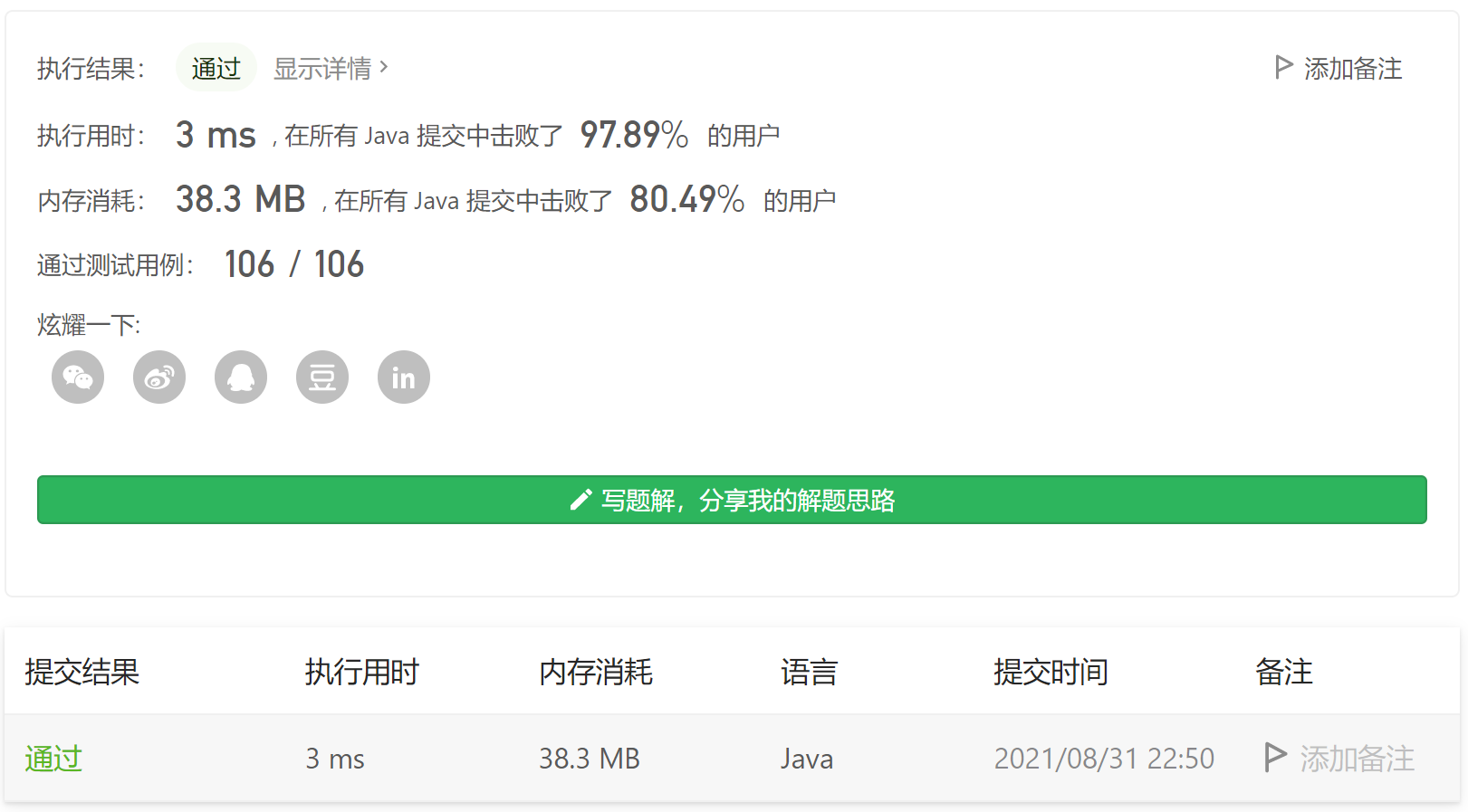展开全文leetcode java
• 求出s1的全排列，然后判断s2中是否包含s1的排列之一 代码如下： class Solution { public: bool checkInclusion(string s1, string s2) { //s2包含s1全排列中的一个 if(s2.size()<s1.size()) return false...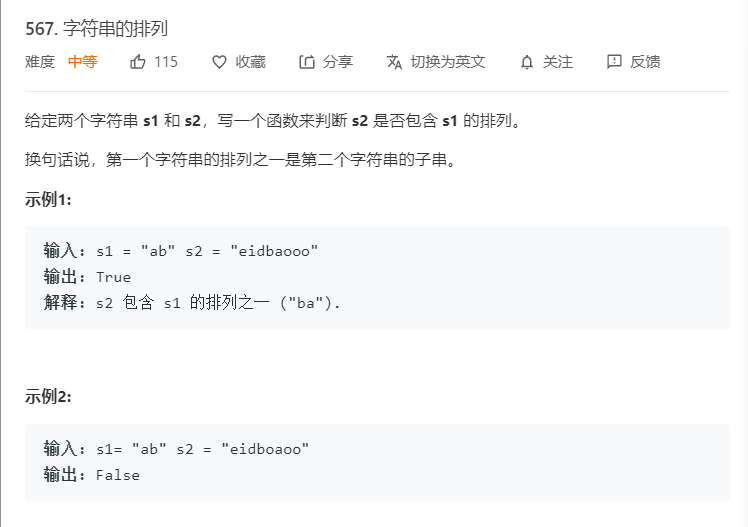1.暴力法

求出s1的全排列，然后判断s2中是否包含s1的排列之一

代码如下：

class Solution {
public:
bool checkInclusion(string s1, string s2) {
//s2包含s1全排列中的一个
if(s2.size()<s1.size())
return false;
if(s2.find(s1)!=string::npos)
return true;
//确定s1的全排列
set<string> tem;
dfs(s1,0,tem);
vector<string> res(tem.begin(),tem.end());
for(int i=0;i<res.size();i++)
if(s2.find(res[i])!=string::npos)
return true;
return false;
}
//使用set为了防止s1中存在重复字母
void dfs(string s,int start,set<string>&tem)
{
if(start == s.size() - 1)
{
tem.insert(s);
return;
}
for(int i = start;i < s.size();i++)
{
swap(s[start],s[i]);
dfs(s,start + 1,tem);
swap(s[start],s[i]);
}
}
};

结果，超时

2.滑动窗口

维护一个大小为s1.size()的窗口，使用hashmap1记录字符串s1内的字符情况，hashmap2记录字符串s2中滑动窗口内的字符情况，当hashmap1==hashmap2时，则返回true

否则，取掉滑动窗口的第一个字符，然后统计滑动窗口之后的一个字符

代码如下：

class Solution {
public:
bool checkInclusion(string s1, string s2) {
if(s1.size()>s2.size())
return false;
int len=s1.size();//滑动窗口的大小
vector<int> hashmap1(26,0);
vector<int> hashmap2(26,0);

for(int i=0;i<len;i++)
{
hashmap1[s1[i]-'a']++;
hashmap2[s2[i]-'a']++;
}
for(int i=len;i<s2.size();i++)
{
if(hashmap1==hashmap2)
return true;
//否则，修改滑动窗口的值
hashmap2[s2[i-len]-'a']--;
hashmap2[s2[i]-'a']++;
}
return hashmap2==hashmap1;
}
};

展开全文• 综述： 使用递归求解问题有时往往令人费解，博主对递归也是头痛不已，以下问题，利用递归很容易求解。... 参考资料： 字符串全排列 Leetcode 欢迎大家扫描关注公众号：编程真相，获取更多精彩的编程技术文章！排列 组合 递归 Leetcode
• LeetCode字符串换行力码五月挑战 Leet 代码的 Python 解决方案可能面临挑战。 第 1 天：第一个坏版本 第2天：珠宝和石头 第3天：赎金记录 Day4：补数 第 5 天：s 字符串中的第一个唯一字符 第6天：多数元素 第7天：...
• 给你两个字符串s1和s2，写一个函数来判断s2是否包含s1的排列。 换句话说，s1的排列之一是s2的子串。 https://leetcode-cn.com/problems/permutation-in-string/ 二.代码 public boolean checkInclusion(String...算法 leetcode
• 567. 字符串排列 给定两个字符串 s1 和 s2，写一个函数来判断 s2 是否包含 s1 的排列。 换句话说，第一个字符串排列之一是第二个字符串的子串。 示例1: 输入: s1 = “ab” s2 = “eidbaooo” 输出: True 解释: ...
• 目录 ...请你重新排列字符串 s ，其中第 i 个字符需要移动到 indices[i] 指示的位置。 返回重新排列后的字符串。 示例 1： 输入：s = "codeleet", indices = [4,5,6,7,0,2,1,3] 输出："lee..字符串 c++ leetcode 数据结构
• 字符串排列 题目传送门 参考题解 思路：交换+递归 代码： class Solution { ArrayList<String> res = new ArrayList<>(); public String[] permutation(String S) { if (S == null || S == ...
• leetcode字符串括号level LeetCode LeetCode 解题记录 Go 使用 Go 语言的解题记录 longestCommonPrefix 最长公共前缀 checkInclusion 字符串的排列 multiply 字符串相乘 reverseWords 翻转字符串里的单词 ...
• 字符串题目大多数用滑动窗口法可以解决 3. 无重复字符的最长子串 int lengthOfLongestSubstring(char * s){ int len=strlen(s); if(len==0)return 0; int start=0,end=0; int flag={0}; int maxlen=0; ...
• leetcode字符串排列（滑动窗口） 问题描述：   给你两个字符串 s1 和 s2 ，写一个函数来判断 s2 是否包含 s1 的排列。换句话说，s1 的排列之一是 s2 的 子串 。 示例： 输入：s1 = “ab” s2 = “eidbaooo” ...leetcode 算法
• 字符串排列（中等） || 滑窗技巧实现代码 Git项目地址：LeetCodeUsingC刷题笔记 本篇解法参考了labuladong的C++滑窗模板，并根据C代码实现做了部分调整，相关改变见注释。 实现代码 // "ky" // ...C语言 leetcode 滑动窗口 字符串
• 给定两个字符串 s1 和 s2，写一个函数来判断 s2 是否包含 s1 的排列。 换句话说，第一个字符串排列之一是第二个字符串的子串。 示例1: 输入: s1 = "ab" s2 = "eidbaooo" 输出: ...
• 前面文章，点击下面链接 我的Python教程，不断整理，反复学习 今日，我决定继续更新Python教程，今天就开始了七十一、Python | Leetcode字符串系列（上篇）。 文章目录 字符串 LeetCode 第3题：无重复字符的最长...
• 给定两个字符串 s1 和 s2，写一个函数来判断 s2 是否包含 s1 的排列。 换句话说，第一个字符串排列之一是第二个字符串的子串。 示例1: 输入: s1 = "ab" s2 = "eidbaooo" 输出: True 解释: s2 包含 s1 的排列之一 ...
• 然后列出这串数字能组合出的字符串。 思路很简单，就是简单的枚举，这里讲一下几个注意的点： 1、首先如果使用的操作是ans.push_back(tempans[i]+str[j])这样，那么有个问题，最初的ans是没有成员的，所以会导致...
• 字符串排列 给定两个字符串 s1 和 s2，写一个函数来判断 s2 是否包含 s1 的排列。 换句话说，第一个字符串排列之一是第二个字符串的子串。 示例1: 输入: s1 = “ab” s2 = “eidbaooo” 输出: True 解释: s2 ...
• leetcode字符串排列 题目描述： 给定两个字符串 s1 和 s2，写一个函数来判断 s2 是否包含 s1 的排列。 换句话说，第一个字符串排列之一是第二个字符串的子串。 示例1: 输入: s1 = "ab" s2 = "eidbaooo" 输出: ...字符串 java 数据结构 算法 leetcode
• 换句话说，第一个字符串排列之一是第二个字符串的子串。 示例1: 输入: s1 = "ab" s2 = "eidbaooo" 输出: True 解释: s2 包含 s1 的排列之一 ("ba"). 示例2: 输入: s1= "ab" s2 = "eidboaoo" 输出: False ...
• package main import ( "fmt" ) func permutation(s string) []string { index := 0 data := []byte(s) result := []string{} TraceBack(index, data, &...func TraceBack(x int, data []byte, res *[]...leetcode golang 算法
• } } 三、运行情况 四、刷题总结 Java 字符串与字符数组的相互转化： （1）字符串转化为字符数组： String str="leetcode"; char[] ch=str.toCharArray();//调用toCharArray()方法将字符串转化为字符数组 for(int i=...leetcode 字符串 字符数组
• 给定两个字符串 s1 和 s2，写一个函数来判断 s2 是否包含 s1 的排列。 换句话说，第一个字符串排列之一是第二个字符串的子串。 示例1: 输入: s1 = "ab" s2 = "eidbaooo" 输出: True 解释: s2 包含 s1 的排列之一 ...
• S and T are strings composed of lowercase letters. In S, no letter occurs more than once. S was sorted in some custom order previously. We want to permute the characters of T so that they match the or...
• 具体描述为：输入一个字符串，打印出该字符串中字符的所有排列。你可以以任意顺序返回这个字符串数组，但里面不能有重复元素。  示例： 输入：s = "abc" 输出：["abc","acb","bac","bca","cab","cba"]  这种...leetcode 数据结构 java python 算法...